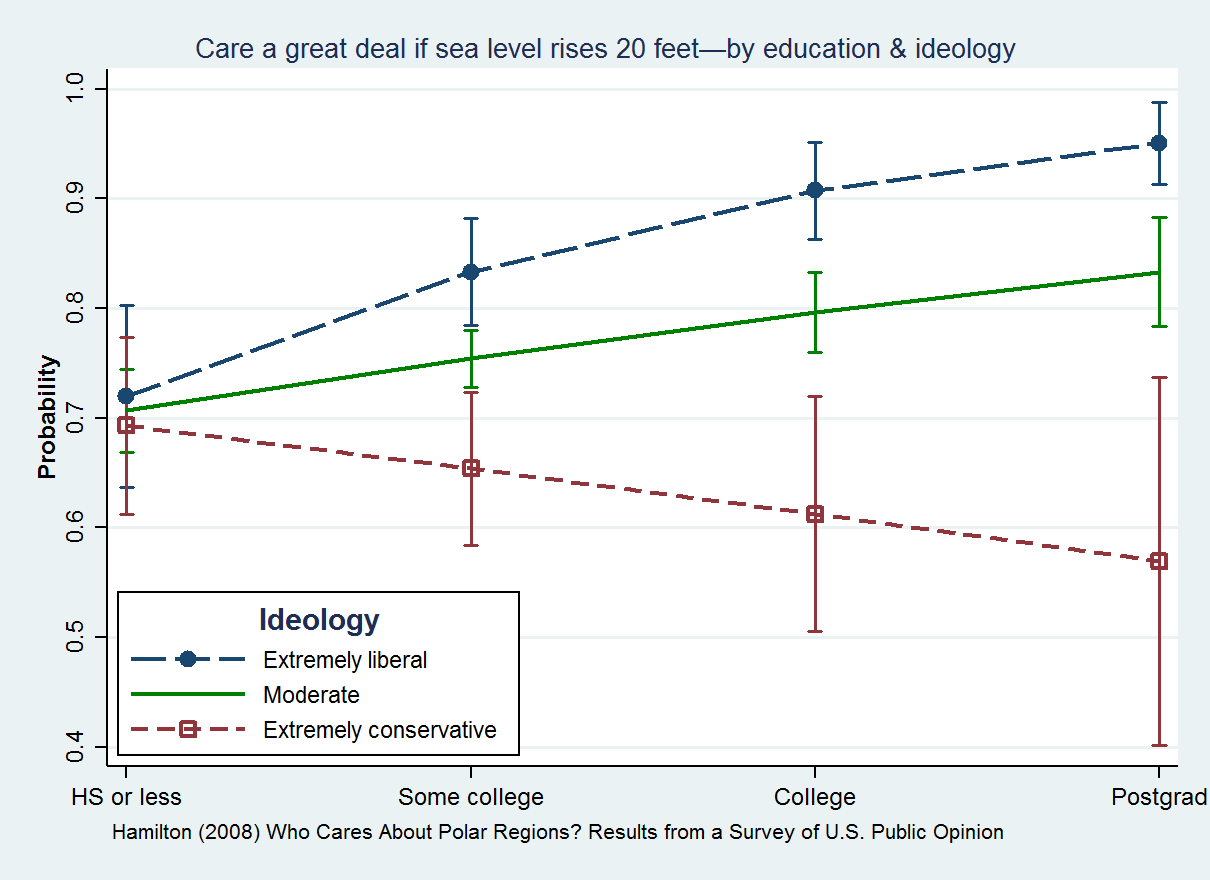# Topic 10 Two-way ANOVA

Before, with one-way ANOVA, we wanted to test the equality of population means for multiple groups.

Now, we want to do something a bit more complicated. We introduce a new categorical variable to our analysis and we want to check if this new variable changes how our initial categorical variable influences the dependent variable. We say we are testing for an interaction between the two categorical variables. I will explain this in more details below.

We use this test to examine the means of one continuous variable (we call it the dependent variable) across different groups defined by two categorical variables (we call them the independent variables).

## 10.1 Interaction between groups

An interaction exists if the effect of one variable X on some other variable Y depends on a third variable Z. This is very abstract. Let me illustrate by using the example below (from Wikipedia)## 10.2 Example

Suppose we are first interested in comparing wages across those with a college degree vs those without a college degree. To test if the mean of wages differs between those with/without college, we simply perform our one-way ANOVA from last lab. Recall that because we only have two categories, this is the same as an independent sample t-test. Suppose that our tests show a significant difference between population means. We will say, therefore, that college degree has an effect on wages.

Now, let us add a new categorical variable: gender. Does that change the effect of college degree on wages? In other words, is there an interactiont between college degree and gender? This is what we want to explore with a two-way ANOVA.

• Null hypothesis: There is an interaction between the variables gender and college degree.
• Alternative hypothesis: There is not interaction between these variables.

## 10.3 Extra

It is not simple to write our null hypothesis. Call Male - M, Female - F, College degree - C, No college degree - N. In the formula below, C M, for example indicates the wage population mean for those with college degree that are males.

• Null hypothesis: (C M - C F) - (N M - N F) = 0
• Alternative hypothesis: (C M - C F) - (N M - N F) $$\neq$$ 0

Note that we are testing both if gender changes the effect of college degree on wages AND if college degree changes the effect of gender on wages.

In words, our null hypothesis is that the variable gender does not influence the effect of college degree on wages AND that the variable regarding college degree does not influence the effect of gender on wages.

## 10.4 Interpretation

If we reject the null ( $$p < 0.05$$ ), we say there is a statistically significant interaction between our categorical variables.

## 10.5 Calculations

The goal for today is no focus on the concepts and interpretation. I will not go over manual calculations for the two-way ANOVA.

## 10.6 Assumptions (same as before)

For the one-way ANOVA test to work, three assumptions need to hold:

1. Normality: The variables of interest should be normally distributed within each group.
• We usually do not perform mathematical tests to check for normality.
• Looking at measures of central tendency and histograms is probably the best way to do so.
1. Independence: There must be no relationship between the variables in each group. These should hold for each categorical variable.
• This is not simple to test.
• Usually, we assume it holds if each group is composed of observations from different units. In other words, there must be no individual that belongs to both groups.
1. Equality of variances: The population variances of all the groups (all possible combinations of our categorical variables) must not be statistically different.
• If variances are equal, we say we have homoskedasticity
• If variances are not equal, we say we have heteroskedasticity
• We can test this!

## 10.7 Things to consider

• You should look at the plot first to check if we have reason to test for an interaction. If the lines are parallel, then we will probably not have a significant interaction.
• You can split file before performing the analysis to separate your results by groups of interest.

## 10.8 Exercise

I will illustrate using the earnings dataset. I will test if the marriage variable mediates the effect of the college variable on wages.

Now, using the “earnings_data.sav” answer the following: does the race variable mediates the effect of college degree on wages? Perform the necessary procedures as I have done above.